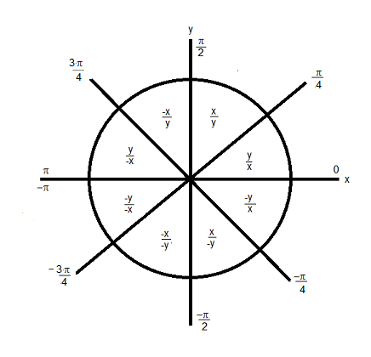# atan2

Four-quadrant inverse tangent of fixed-point values

## Syntax

``z = atan2(y,x)``

## Description

example

````z = atan2(y,x)` returns the four-quadrant arctangent of `fi` inputs `y` and `x`.```

## Examples

collapse all

Use the `atan2` function to calculate the arctangent of unsigned and signed fixed-point input values.

Unsigned Input Values

This example uses unsigned, 16-bit word length values.

```y = fi(0.125,0,16); x = fi(0.5,0,16); z = atan2(y,x)```
```z = 0.2450 DataTypeMode: Fixed-point: binary point scaling Signedness: Unsigned WordLength: 16 FractionLength: 15 ```

Signed Input Values

This example uses signed, 16-bit word length values.

```y = fi(-0.1,1,16); x = fi(-0.9,1,16); z = atan2(y,x)```
```z = -3.0309 DataTypeMode: Fixed-point: binary point scaling Signedness: Signed WordLength: 16 FractionLength: 13 ```

## Input Arguments

collapse all

y-coordinates, specified as a scalar, vector, matrix, or multidimensional array.

`y` and `x` can be real-valued, signed or unsigned scalars, vectors, matrices, or `N`-dimensional arrays containing fixed-point angle values in radians. The inputs `y` and `x` must be the same size. If they are not the same size, at least one input must be a scalar value. Valid data types of `y` and `x` are:

• `fi` single

• `fi` double

• `fi` fixed-point with binary point scaling

• `fi` scaled double with binary point scaling

Data Types: `fi`

x-coordinates, specified as a scalar, vector, matrix, or multidimensional array.

`y` and `x` can be real-valued, signed or unsigned scalars, vectors, matrices, or `N`-dimensional arrays containing fixed-point angle values in radians. The inputs `y` and `x` must be the same size. If they are not the same size, at least one input must be a scalar value. Valid data types of `y` and `x` are:

• `fi` single

• `fi` double

• `fi` fixed-point with binary point scaling

• `fi` scaled double with binary point scaling

Data Types: `fi`

## Output Arguments

collapse all

Four-quadrant arctangent, returned as a scalar, vector, matrix, or multidimensional array.

`z` is the four-quadrant arctangent of `y` and `x`. The `numerictype` of `z` depends on the signedness of `y` and `x`:

• If either `y` or `x` is signed, then `z` is a signed, fixed-point number in the range [–pi,pi]. It has a 16-bit word length and 13-bit fraction length (`numerictype(1,16,13)`).

• If both `y` and `x` are unsigned, then `z` is an unsigned, fixed-point number in the range [0,pi/2]. It has a 16-bit word length and 15-bit fraction length (`numerictype(0,16,15)`).

The output, `z`, is always associated with the default `fimath`.

collapse all

The four-quadrant arctangent is defined as follows, with respect to the `atan` function:

## Algorithms

collapse all

The `atan2` function computes the four-quadrant arctangent of fixed-point inputs using an 8-bit lookup table as follows:

1. Divide the input absolute values to get an unsigned, fractional, fixed-point, 16-bit ratio between 0 and 1. The absolute values of y and x determine which value is the divisor.

The signs of the `y` and `x` inputs determine in what quadrant their ratio lies. The input with the larger absolute value is used as the denominator, thus producing a value between 0 and 1.2. Compute the table index, based on the 16-bit, unsigned, stored integer value:

1. Use the 8 most-significant bits to obtain the first value from the table.

2. Use the next-greater table value as the second value.

3. Use the 8 least-significant bits to interpolate between the first and second values using nearest neighbor linear interpolation. This interpolation produces a value in the range [0, pi/4).

4. Perform octant correction on the resulting angle, based on the values of the original `y` and `x` inputs.

This arctangent calculation is accurate only to within the top 16 most-significant bits of the input.

### fimath Propagation Rules

The `atan2` function ignores and discards any `fimath` attached to the inputs. The output, `z`, is always associated with the default `fimath`.

## Version History

Introduced in R2012a